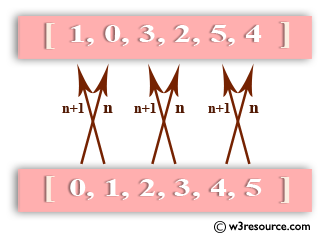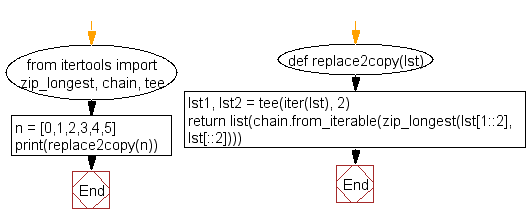﻿ Python: Change the position of every n-th value with the (n+1)th in a list - w3resource# Python: Change the position of every n-th value with the (n+1)th in a list

## Python List: Exercise - 38 with Solution

Write a Python program to change the position of every n-th value with the (n+1)th in a list.Sample Solution:-

Python Code:

``````from itertools import zip_longest, chain, tee
def replace2copy(lst):
lst1, lst2 = tee(iter(lst), 2)
return list(chain.from_iterable(zip_longest(lst[1::2], lst[::2])))
n = [0,1,2,3,4,5]
print(replace2copy(n))
```
```

Sample Output:

```[1, 0, 3, 2, 5, 4]
```

Flowchart:## Visualize Python code execution:

The following tool visualize what the computer is doing step-by-step as it executes the said program:

Python Code Editor:

Have another way to solve this solution? Contribute your code (and comments) through Disqus.

What is the difficulty level of this exercise?

Test your Python skills with w3resource's quiz

﻿

## Python: Tips of the Day

Floor Division:

When we speak of division we normally mean (/) float division operator, this will give a precise result in float format with decimals.

For a rounded integer result there is (//) floor division operator in Python. Floor division will only give integer results that are round numbers.

```print(1000 // 300)
print(1000 / 300)```

Output:

```3
3.3333333333333335```